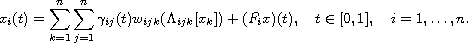Electron. J. Diff. Equ., Vol. 2014 (2014), No. 71, pp. 1-17.

### Existence of solutions for an n-dimensional operator equation and applications to BVPs George L. Karakostas

Abstract:
By applying the Guo-Lakshmikantham fixed point theorem on high dimensional cones, sufficient conditions are given to guarantee the existence of positive solutions of a system of equations of the formApplications are given to three boundary value problems: A 3-dimensional 3+3+3 order boundary value problem with mixed nonlocal boundary conditions, a 2-dimensional 2+4 order nonlocal boundary value problem discussed in , and a 2-dimensional 2+2 order nonlocal boundary value problem discussed in . In the latter case we provide some fairly simpler conditions according to those imposed in .

Submitted February 19, 2014. Published March 16, 2014.
Math Subject Classifications: 34B10, 34K10.
Key Words: Krasnoselskii's fixed point theorem; high-dimensional cones; nonlocal and multipoint boundary value problems; system of differential equations.

Show me the PDF file (270 KB), TEX file, and other files for this article.George L. Karakostas Department of Mathematics University of Ioannina 451 10 Ioannina, Greece email: gkarako@uoi.gr